# Balaraju Akula SA (Maths), Zphs Babilgaon

•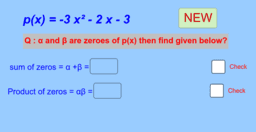### Assessment form of quadratic and cubic polynomials

Activity

Balaraju Akula SA (Maths), Zphs Babilgaon

•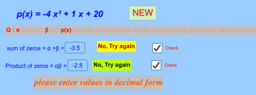### (వర్గ మరియు ఘన బహుపదుల యొక్క శూన్యాలు )

Activity

Balaraju Akula SA (Maths), Zphs Babilgaon

•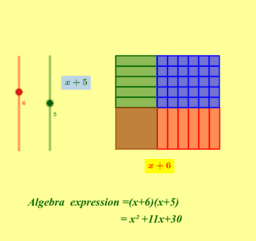### Algebra tiles

Activity

Balaraju Akula SA (Maths), Zphs Babilgaon

•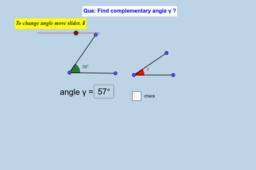### Complementary angle Assessment form

Activity

Balaraju Akula SA (Maths), Zphs Babilgaon

•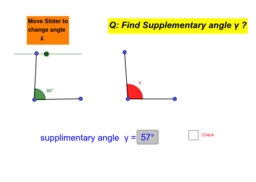### Supplementary angle assessment form

Activity

Balaraju Akula SA (Maths), Zphs Babilgaon

•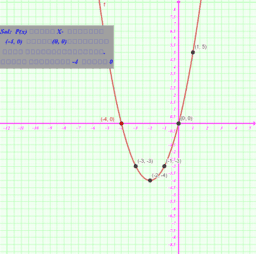### quadratic polynomial (వర్గ బహుపది )

Activity

Balaraju Akula SA (Maths), Zphs Babilgaon

•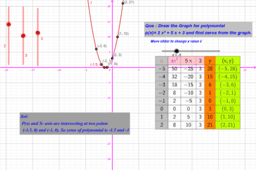### Graphical representation of quadratic polynomial

Activity

Balaraju Akula SA (Maths), Zphs Babilgaon

•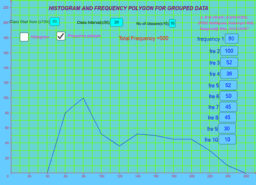### Histogram and frequency polygon for grouped Data

Activity

Balaraju Akula SA (Maths), Zphs Babilgaon

•### Formative Assessment in sets

Activity

Balaraju Akula SA (Maths), Zphs Babilgaon

•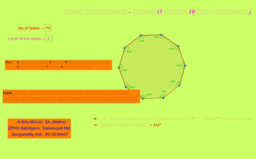### Types of regular polygons (క్రమ బహుభుజులు - రకాలు )

Activity

Balaraju Akula SA (Maths), Zphs Babilgaon

•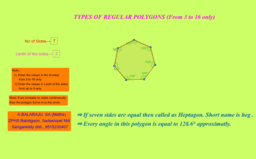### Types of regular polygons

Activity

Balaraju Akula SA (Maths), Zphs Babilgaon

•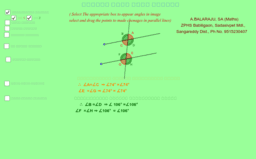Activity

Balaraju Akula SA (Maths), Zphs Babilgaon

•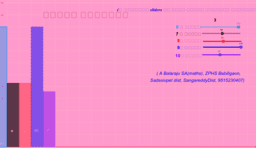### Histogram (Sopana chitram)

Activity

Balaraju Akula SA (Maths), Zphs Babilgaon

•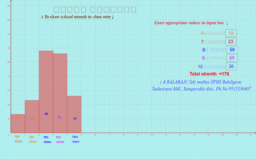### Sopana chitramu(సోపాన చిత్రము )

Activity

Balaraju Akula SA (Maths), Zphs Babilgaon

•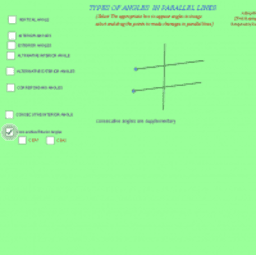### Types of Angles in parallel lines

Activity

Balaraju Akula SA (Maths), Zphs Babilgaon

•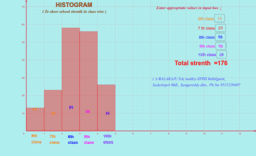### HISTOGRAM 2

Activity

Balaraju Akula SA (Maths), Zphs Babilgaon

•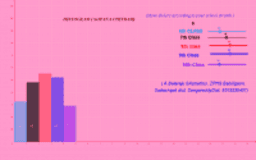### Histogram

Activity

Balaraju Akula SA (Maths), Zphs Babilgaon

•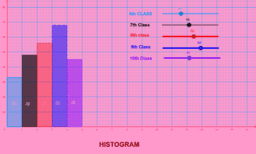### HISTOGRAM (SOPANA CHITRAM)

Activity

Balaraju Akula SA (Maths), Zphs Babilgaon